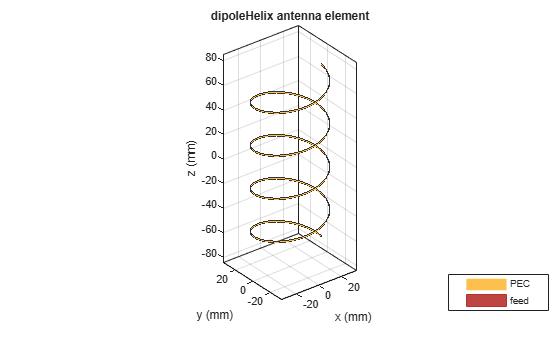# dipoleHelix

Create helical dipole antenna

## Description

The `dipoleHelix ` object is a helical dipole antenna. The antenna is typically center-fed. You can move the feed along the antenna length using the feed offset property. Helical dipoles are used in satellite communications and wireless power transfers.

The width of the strip is related to the diameter of an equivalent cylinder by this equation

`$w=2d=4r$`

where:

• w is the width of the strip.

• d is the diameter of an equivalent cylinder.

• r is the radius of an equivalent cylinder.

For a given cylinder radius, use the `cylinder2strip` utility function to calculate the equivalent width. The default helical dipole antenna is center-fed. The circular ground plane is on the X-Y plane. Commonly, helical dipole antennas are used in axial mode. In this mode, the helical dipole circumference is comparable to the operating wavelength, and has maximum directivity along its axis. In normal mode, the helical dipole radius is small compared to the operating wavelength. In this mode, the helical dipole radiates broadside, that is, in the plane perpendicular to its axis. The basic equation for the helical dipole antenna is:

$\begin{array}{l}x=r\mathrm{cos}\left(\theta \right)\\ y=r\mathrm{sin}\left(\theta \right)\\ z=S\theta \end{array}$

where:

• r is the radius of the helical dipole.

• θ is the winding angle.

• S is the spacing between turns.

For a given pitch angle in degrees, use the `helixpitch2spacing` utility function to calculate the spacing between the turns in meters.## Creation

### Syntax

``dh = dipoleHelix``
``dh = dipoleHelix(Name,Value)``

### Description

example

``` `dh = dipoleHelix` creates a helical dipole antenna. The default antenna operates around 2 GHz.```

example

``` `dh = dipoleHelix(Name,Value)` creates a helical dipole antenna, with additional properties specified by one or more name–value pair arguments. `Name` is the property name and `Value` is the corresponding value. You can specify several name-value pair arguments in any order as `Name1`, `Value1`, `...`, `NameN`, `ValueN`. Properties not specified retain their default values.```

## Properties

expand all

Turn radius, specified as a scalar in meters.

Example: `'Radius',2`

Data Types: `double`

Strip width, specified as a scalar in meters.

### Note

Strip width should be less than `'Radius'`/5 and greater than `'Radius'`/250. 

Example: `'Width',5`

Data Types: `double`

Number of turns of the helical dipole, specified a scalar.

Example: `'Turns',2`

Data Types: `double`

Spacing between turns, specified as a scalar in meters.

Example: `'Spacing',1.5`

Data Types: `double`

Direction of helical dipole turns (windings), specified as `'CW'` or `'CCW'`.

Example: `'WindingDirection'`,`'CW'`

Data Types: `char` | `string`

Lumped elements added to the antenna feed, specified as a lumped element object handle. For more information, see `lumpedElement`.

Example: `'Load',lumpedelement`. `lumpedelement` is the object handle for the load created using `lumpedElement`.

Example: ```dh.Load = lumpedElement('Impedance',75)```

Signed distance from center along length and width of ground plane, specified as a two-element vector in meters. Use this property to adjust the location of the feedpoint relative to the ground plane and patch.

Example: `'FeedOffset',[0.01 0.01]`

Data Types: `double`

Tilt angle of the antenna, specified as a scalar or vector with each element unit in degrees. For more information, see Rotate Antennas and Arrays.

Example: `'Tilt',90`

Example: `ant.Tilt = 90`

Example: `'Tilt',[90 90]`,`'TiltAxis',[0 1 0;0 1 1]` tilts the antenna at 90 degrees about the two axes, defined by vectors.

### Note

The `wireStack` antenna object only accepts the dot method to change its properties.

Data Types: `double`

Tilt axis of the antenna, specified as:

• Three-element vectors of Cartesian coordinates in meters. In this case, each vector starts at the origin and lies along the specified points on the X-, Y-, and Z-axes.

• Two points in space, each specified as three-element vectors of Cartesian coordinates. In this case, the antenna rotates around the line joining the two points in space.

• A string input describing simple rotations around one of the principal axes, 'X', 'Y', or 'Z'.

Example: `'TiltAxis',[0 1 0]`

Example: `'TiltAxis',[0 0 0;0 1 0]`

Example: `ant.TiltAxis = 'Z'`

### Note

The `wireStack` antenna object only accepts the dot method to change its properties.

## Object Functions

 `show` Display antenna or array structure; display shape as filled patch `info` Display information about antenna or array `axialRatio` Axial ratio of antenna `beamwidth` Beamwidth of antenna `charge` Charge distribution on metal or dielectric antenna or array surface `current` Current distribution on metal or dielectric antenna or array surface `design` Design prototype antenna or arrays for resonance at specified frequency `EHfields` Electric and magnetic fields of antennas; Embedded electric and magnetic fields of antenna element in arrays `impedance` Input impedance of antenna; scan impedance of array `mesh` Mesh properties of metal or dielectric antenna or array structure `meshconfig` Change mesh mode of antenna structure `pattern` Radiation pattern and phase of antenna or array; Embedded pattern of antenna element in array `patternAzimuth` Azimuth pattern of antenna or array `patternElevation` Elevation pattern of antenna or array `returnLoss` Return loss of antenna; scan return loss of array `sparameters` S-parameter object `vswr` Voltage standing wave ratio of antenna

## Examples

collapse all

Create a default helical dipole antenna and view it.

`dh = dipoleHelix`
```dh = dipoleHelix with properties: Radius: 0.0220 Width: 1.0000e-03 Turns: 3 Spacing: 0.0350 WindingDirection: 'CCW' FeedOffset: 0 Tilt: 0 TiltAxis: [1 0 0] Load: [1x1 lumpedElement] ```
`show(dh)`Create a four-turn helical dipole antenna with a turn radius of 28 mm and a strip width of 1.2 mm.

```dh = dipoleHelix('Radius',28e-3,'Width',1.2e-3,'Turns',4); show(dh)```Plot the radiation pattern of the helical dipole at 1.8 GHz.

`pattern(dh, 1.8e9);`Balanis, C. A. Antenna Theory. Analysis and Design. 3rd Ed. Hoboken, NJ: John Wiley & Sons, 2005.

 Volakis, John. Antenna Engineering Handbook. 4th Ed. New York: McGraw-Hill, 2007.